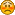Hello Guest

# [Solved] Out of memory : glDrawElements

• 1 Replies
• 4541 Views####Rpahut

•• 2##### [Solved] Out of memory : glDrawElements
« on: March 27, 2011, 18:37:54 »
Okay, seems like I cannot figure it out myself.

I'm (unsuccessfuly) trying to use VBO's to display bunch of points.

First I was trying to follow this article and all I got was clear black screen. Then I decided to try lwjgl_debug library and it rewarded me with Out of memory exception on call to glDrawRangeElements.

So I've found this source and tried different approach, again with the same result - blank screen with the normal library, Out of memory at glDrawElements with debug library.

As I see it, 49152 bytes of vertex data is not quite enough to run out of memory, so I believe I'm just doing something wrong. I'm not sure I completely understand how buffer objects and buffers related to each other, and thus where problem can be. Any help is appreciated.

Here goes the code:
Code: [Select]
`    // after GL init    // create and store VBO id for vertex buffer    IntBuffer ib = BufferUtils.createIntBuffer(1);    ARBVertexBufferObject.glGenBuffersARB(ib);    coordsVBOID = ib.get(0);    // create and store VBO id for index buffer    ib = BufferUtils.createIntBuffer(1);    ARBVertexBufferObject.glGenBuffersARB(ib);    indexVBOID = ib.get(0);    // gen vertex data    FvertexBuffer = BufferUtils.createFloatBuffer(16*16*16*3);    for (int by = 0; by < 16; by++) {       for (int bx = 0; bx < 16; bx++) {           for (int bz = 0; bz < 16; bz++) {               FvertexBuffer.put(2.f * bx);               FvertexBuffer.put(2.f * by);               FvertexBuffer.put(2.f * bz);          }       }    }    // send vertex data to VRAM    ARBVertexBufferObject.glBindBufferARB(ARBVertexBufferObject.GL_ARRAY_BUFFER_ARB, coordsVBOID);    ARBVertexBufferObject.glBufferDataARB(ARBVertexBufferObject.GL_ARRAY_BUFFER_ARB, FvertexBuffer, ARBVertexBufferObject.GL_STATIC_DRAW_ARB);    // gen index data    IindexBuffer = BufferUtils.createIntBuffer(16*16*16);    for (int i = 0; i < 16*16*16; i++) {       IindexBuffer.put(i);    }    // send index data to VRAM    ARBVertexBufferObject.glBindBufferARB(ARBVertexBufferObject.GL_ELEMENT_ARRAY_BUFFER_ARB, coordsVBOID);    ARBVertexBufferObject.glBufferDataARB(ARBVertexBufferObject.GL_ELEMENT_ARRAY_BUFFER_ARB, IindexBuffer, ARBVertexBufferObject.GL_STATIC_DRAW_ARB);`
Code: [Select]
`    // render    GL11.glEnableClientState(GL11.GL_VERTEX_ARRAY);    ARBVertexBufferObject.glBindBufferARB(ARBVertexBufferObject.GL_ARRAY_BUFFER_ARB, coordsVBOID);    GL11.glVertexPointer(3, GL11.GL_FLOAT, 0, 0);    ARBVertexBufferObject.glBindBufferARB(ARBVertexBufferObject.GL_ELEMENT_ARRAY_BUFFER_ARB, indexVBOID);    GL12.glDrawRangeElements(GL11.GL_POINTS, 0, 16*16*16, 16*16*16, GL11.GL_UNSIGNED_INT, 0); // Out of memory    ARBVertexBufferObject.glBindBufferARB(ARBVertexBufferObject.GL_ARRAY_BUFFER_ARB, 0);    GL11.glDisableClientState(GL11.GL_VERTEX_ARRAY);`
Thanks.
« Last Edit: March 27, 2011, 22:19:49 by Rpahut »####Rpahut

•• 2##### Re: Out of memory : glDrawElements
« Reply #1 on: March 27, 2011, 22:11:16 »
Oh, I've finally got it to work. I feel almost as accomplished as Alexander Makeonian when he conquered Babylon.

I still have no idea where I was wrong before. Looks like index data upload before rendering is the missing partHere is the working code:
Code: [Select]
`import java.nio.FloatBuffer;import java.nio.IntBuffer;import org.lwjgl.BufferUtils;import org.lwjgl.opengl.ARBVertexBufferObject;public class VBOUtils {    static int CreateVBOID() {        IntBuffer buf = BufferUtils.createIntBuffer(1);        ARBVertexBufferObject.glGenBuffersARB(buf);        return buf.get(0);    }    static void DeleteVBOID(int vboID) {        ARBVertexBufferObject.glDeleteBuffersARB(vboID);    }    static void SetBufferData(int vboID, FloatBuffer fbuf) {        ARBVertexBufferObject.glBindBufferARB(ARBVertexBufferObject.GL_ARRAY_BUFFER_ARB, vboID);        ARBVertexBufferObject.glBufferDataARB(ARBVertexBufferObject.GL_ARRAY_BUFFER_ARB, fbuf, ARBVertexBufferObject.GL_STATIC_DRAW_ARB);    }    static void SetBufferData(int vboID, IntBuffer ibuf) {        ARBVertexBufferObject.glBindBufferARB(ARBVertexBufferObject.GL_ELEMENT_ARRAY_BUFFER_ARB, vboID);        ARBVertexBufferObject.glBufferDataARB(ARBVertexBufferObject.GL_ELEMENT_ARRAY_BUFFER_ARB, ibuf, ARBVertexBufferObject.GL_STATIC_DRAW_ARB);    }    static int GetDataSize(int vboID, int target) {        ARBVertexBufferObject.glBindBufferARB(target, vboID);        return ARBVertexBufferObject.glGetBufferParameterARB(target, ARBVertexBufferObject.GL_BUFFER_SIZE_ARB);    }    static void Unbind(int target) {        ARBVertexBufferObject.glBindBufferARB(target, 0);    }}`
Code: [Select]
`        // initialisation        // create and store VBO id's for buffers        coordsVBOID = VBOUtils.CreateVBOID();        indexVBOID = VBOUtils.CreateVBOID();        // gen vertex data        FvertexBuffer = BufferUtils.createFloatBuffer(16 * 16 * 16 * 3);        for (int by = 0; by < 16; by++) {            for (int bx = 0; bx < 16; bx++) {                for (int bz = 0; bz < 16; bz++) {                    FvertexBuffer.put(2.f * bx);                    FvertexBuffer.put(2.f * by);                    FvertexBuffer.put(2.f * bz);                }            }        }        FvertexBuffer.flip();        // send vertex data to VRAM        VBOUtils.SetBufferData(coordsVBOID, FvertexBuffer);        int dataSize = VBOUtils.GetDataSize(coordsVBOID, ARBVertexBufferObject.GL_ARRAY_BUFFER_ARB);        System.out.println("Vertex data size:");        System.out.println(dataSize);        // gen index data        IindexBuffer = BufferUtils.createIntBuffer(16 * 16 * 16);        for (int i = 0; i < 16 * 16 * 16; i++) {            IindexBuffer.put(i);        }        IindexBuffer.flip();        // send index data to VRAM        VBOUtils.SetBufferData(indexVBOID, IindexBuffer);        ARBVertexBufferObject.glBindBufferARB(ARBVertexBufferObject.GL_ELEMENT_ARRAY_BUFFER_ARB, indexVBOID);        dataSize = VBOUtils.GetDataSize(ARBVertexBufferObject.GL_ELEMENT_ARRAY_BUFFER_ARB, indexVBOID);        System.out.println("Index data size:");        System.out.println(dataSize);`
Code: [Select]
`        // rendering        ARBVertexBufferObject.glBindBufferARB(ARBVertexBufferObject.GL_ARRAY_BUFFER_ARB, coordsVBOID);        // map - edit data - unmap here        //ARBVertexBufferObject.glMapBufferARB(...);        glVertexPointer(3, GL_FLOAT, 0, 0);        VBOUtils.SetBufferData(indexVBOID, IindexBuffer);        glEnableClientState(GL_VERTEX_ARRAY);        glDrawElements(GL_POINTS, 16*16*16, GL_UNSIGNED_INT, 0);        VBOUtils.Unbind(ARBVertexBufferObject.GL_ARRAY_BUFFER_ARB);        VBOUtils.Unbind(ARBVertexBufferObject.GL_ELEMENT_ARRAY_BUFFER_ARB);`